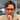# Repeating-key XOR II

June 20, 2021

Building up on our previous repeating-key-xor post we will be tackling the exact same problem, but we will assume this time that we do not know the length of our `key`.

Before we jump into solution mode we will need to figure out how to compute the edit distance, also called Hamming distance, between two strings. This is nothing more than computing the number of differing bits (not bytes) between those strings. This value will help us determine whether we are guessing a good key length or not in the following section.

This can be done by XORing characters of both strings and counting the number of `1` bits in the result. There are two ways that I know of to display the bit representation of a string in `Ruby`:

1. Using `ord.to_s(2)`
• `'a'.ord.to_s(2)` => `"1100001"`
2. Using `.unpack('B*')`
• `'a'.unpack('B*')` => `["01100001"]`

So let’s see the hamming distance between the strings `ha` and `co`.

`ha` => `[h, o]` => `["1101000", "1100001"]`
`co` => `[c, o]` => `["1100011", "1101111"]`

Between `h` and `c` we have `3` bits that are different.
Between `a` and `o` we also have `3` bits that are different.

Given that, the Hamming distance between those two strings is `6`.

Let’s automate that using `Ruby`.

```def hamming(s1, s2) raise 'Both strings should have the same length' if s1.size != s2.size s1.size.times.reduce(0) do |total, index| xor_result = s1[index].ord ^ s2[index].ord total += xor_result.to_s(2).count('1') end end puts hamming('ha', 'co') # 6```

With that out of the way we can start working on figuring out the key length.

## Figuring out the key length

The intuition here is that the Hamming distance between `blocks` XORed by the same key should be relatively low when compared to blocks XORed by different keys. Intuitively this makes sense since we will have less differing bits in average.

If the statement above is confusing it helps to remember that XORing equal strings yields only zero bits and XORing similar strings yields a low number, which corresponds to a low number of 1s in its binary representation. Investigating XOR properties might help with the intuition.

Now let’s see what we mean by `blocks` in our initial statement with a visual example:

Buffer: `THIS_MESSAGE_IS_UNREADABLE!_:)`
Key: `SECRET`

This message will be XORed like:

```T|H|I|S|_|M|E|S|S|A|G|E|_|I|S|_|U|N|R|E|A|D|A|B|L|E|!|_|:|)| S|E|C|R|E|T|S|E|C|R|E|T|S|E|C|R|E|T|S|E|C|R|E|T|S|E|C|R|E|T| -----------|-----------|-----------|-----------|-----------| --BLOCK 1--|--BLOCK 2--|--BLOCK 3--|--BLOCK 4--|--BLOCK 5--|```

So each `block` has `KEY size` characters, in this case 5 characters since that’s the length of our KEY.

Ultimately we will still need to guess our key length. All this algorithm really does is to provide us with a few good guesses so we can run the algorithm detailed in our previous post with the best guesses that we generate.

Let’s formalize our algorithm:

1. Iterate through `KEY sizes` from 2 to 40 (we are assuming our keys have at maximum 40 bytes in this example)
2. For each `KEY size`:
• take the first `KEY size` worth of bytes (first block) from our encoded message
• take the second `KEY size` worth of bytes (second block) from our encoded message
• compute the hamming distance between these two
• normalize this result by dividing by the `KEY size`.
3. Repeat step 2 with the second and third `KEY size` worth of bytes (second and third blocks).
4. Repeat step 2 with the third and fourth `KEY size` worth of bytes (third and fourth blocks).
5. Sum the values from steps two to four and divide the result by three (averaging the result).
6. Store the result somewhere
7. After the score for every `KEY size` is calculated, return the lowest three values.

This is one of the cases where reading the source code is actually easier than reading the specification, so let’s implement it in `Ruby`:

```# Trying keys between 2 and 40 characters KEY_LEN_RANGE = (2..40) Key = Struct.new(:distance, :len, keyword_init: true) do def key_sort(other) distance <=> other.distance end end def find_key_candidates(buffer) # Step 1 and Step 6 (storing in an Array) keys = KEY_LEN_RANGE.map do |key_len| c1 = buffer.slice(0, key_len) c2 = buffer.slice(key_len, key_len) c3 = buffer.slice(key_len * 2, key_len) c4 = buffer.slice(key_len * 3, key_len) # Step 2 d1 = hamming(c1, c2) / key_len.to_f # Step 3 d2 = hamming(c2, c3) / key_len.to_f # Step 4 d3 = hamming(c3, c4) / key_len.to_f # Step 5 distance = (d1 + d2 + d3) / 3.0 Key.new(distance: distance, len: key_len) end # Step 7 keys.sort(&:key_sort).first(3) end```

## What now?

Now we can run the algorithm implemented in our previous post and output the results to see which one yields the best decryption.

We should run it with a small modification, which is:

```candidates = find_key_candidates(encoded_message) keys = candidates.map do |candidate| # Algorithm from previous post end decoded_results = keys.map do |key| repeating_key_xor(encoded_message, key.bytes).pack('C*') end puts decoded_results.inspect # We can also output the result that resembles English the most. puts decoded_results.max_by { |r| english_score(r) }```

And we have reached the end of our exercise! By this point we should have all the tools we need to break the repeating-key XOR algorithm regardless if we know the key length or not.Bernardo de Araujo

Application Security Manager @Shopify.